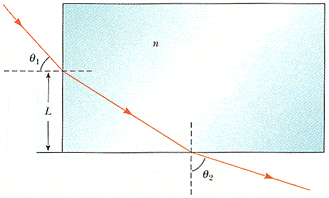# Snell's Law - figuring out index of refraction

Munky
Snell's Law -- figuring out index of refraction

## Homework Statement

A light ray enters a rectangular block of plastic at an angle of θ1 = 45.0° and emerges at an angle of θ2 = 79.0°, as shown in Figure P35.71.

(the image is of light entering the block from the west, and exiting from the south -- it is NOT exiting parallel sides, but perpendicular sides)

Determine the index of refraction of the plastic.

n= index of refraction of block of plastic

## Homework Equations

sin (angle of incident) = n sin (angle of refraction)

## The Attempt at a Solution

I know that some kind of trig relationship is involved here, but I can't identify it.

I have two equations with two unknowns.

First angle of incidence is 45deg, giving

sin 45deg = n sin (angle of refraction)

Second angle is 79deg, giving

n sin (angle of incedence) = sin 79deg

I can solve for n and substitute, but then i end up with one equation and two unknowns (the internal angles).

I can't solve for an angle, taking the inverse sin of an unknown n.

Any help?

thx,
munky

Mentor

You need to relate the angle of refraction to the angle of incidence. Draw yourself a diagram. Hint: Look for the right triangle.

Munkyhere's the image.

all i can gather from the right angle formed by the norms is that the internal angles also add up to 90deg. The only way the external angles relate to the internal angles, though, is by n... which i don't know...

Mentor

all i can gather from the right angle formed by the norms is that the internal angles also add up to 90deg.
That's the key. If the two internal angles add to 90, how do their trig functions (sine, cosine) relate?
The only way the external angles relate to the internal angles, though, is by n... which i don't know...
First relate the internal angles (or trig functions of them) to each other, then use that relationship in your Snell's law equations. (You'll end up with two equations and two unknowns--perfect.)

Munky

I'm getting ready to crunch the numbers now, but I think I've found it... I knew it was some trig thing... it's literally been almost twenty years since I've done trig... lol.

What I found is that in a right triangle, the sin of an angle is equal to the cosine of it's compliment. So it should follow that the internal refraction and internal incedence angles are the sin and cos of the same angle... due to their complimentary nature?

Munky

As my daughter is fond of saying... "Waaaahooo!"
Thanks for your time. Now, according to Murphy, since I spent so much time on that, I won't need to know it tomorrow... lol.

Mentor

What I found is that in a right triangle, the sin of an angle is equal to the cosine of it's compliment. So it should follow that the internal refraction and internal incedence angles are the sin and cos of the same angle... due to their complimentary nature?
You've got it:
sinθa = cosθb

Now, according to Murphy, since I spent so much time on that, I won't need to know it tomorrow... lol.
Ain't that always how it is?